U.S. Department of Transportation
1200 New Jersey Avenue, SE
Washington, DC 20590
202-366-4000

Federal Highway Administration Research and Technology
Coordinating, Developing, and Delivering Highway Transportation InnovationsThis report is an archived publication and may contain dated technical, contact, and link information
 Federal Highway Administration > Publications > Research Publications > LTPP Publications > 03093 > Appendb.Cfm Study of Long-Term Pavement Performance (LTPP): Pavement Deflections
Publication Number: FHWA-RD-03-093
Date: August 2006

# Study of Long-Term Pavement Performance (LTPP): Pavement Deflections

## Appendix B. Semiautomatic Slic Procedure for FWD Data Screening

The original SLIC method for finding sensor-positioning errors was a manual-visual examination of the transformed plot of a deflection basin. This visual method graph plotted the natural logarithm of the negative of the natural logarithm of the normalized deflections (on the vertical y-axis) versus the natural logarithm of the sensor position (on the horizontal x-axis). These graphs were very close to linear, but often with a slight downward concavity, when the sensor positions were correctly reported. The SLIC graphs shown in appendix C through L show marked departure from this typical pattern when sensors are not positioned as reported.

The first attempt at automating the detection of sensor-positioning errors used multiple linear regression to fit a quadratic curve (second degree polynomial) of ln(offset), y, to ln(-ln(normalized deflection)), x. The reason for reversing the axes was that we wanted to predict the value of the ln (offset) from the resulting second-degree equation. To try to go the other direction was sometimes impossible because the quadratic equation that was fit, a concave down parabola, might not reach the y-value corresponding to sensor 7. There was also too much bias and variation in this method when the equation could be solved.

The regression would always utilize the center deflection for normalizing, and five other points, but would leave out the point corresponding to the sensor in question. Then the position of the sensor in question would be predicted from its (normalized) deflection using the best-fitting curve to the other points. The predicted values of the sensor position would be plotted as a time series using all the test dates for one FWD. Then the plots would be examined (visually) for any consistent sequence of unusual values. This method was successful at finding the errors previously reported. However, there was a bias in the predicted results for sensor 7, and a model that eliminated that bias was found. The newer model also reduced the variation in the predicted results. This newer version used a model of the form shown in figure 7 (¦nd¦represents normalized deflection):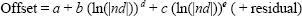Figure 7. Equation. Offset, with exponents d and e.

The exponents, d and e, had to be selected before running any multiple regression. A dataset where no previous errors had been found was used to select the values of d and e. A program was written to try many values of d and e and select the values that predicted a particular sensor with little or no bias and the least variation. For the prediction of sensor 7, for example, these values for d and e are 0.6 and 2.2, respectively. The procedure also examined how many sensors to use, and the optimal number for sensor 7 was four, using the points corresponding to sensors 3 through 6. Apparently the simple curves investigated are not quite adequate to describe the entire (transformed) deflection basin. To estimate where a particular sensor was on a given test date, the entire set of data for that day was averaged, and then a multiple linear regression was run. For example, to estimate where sensor 7 on FWD number 131 was positioned on August 12, 1998, the average deflections on that day were calculated as shown in figure 8.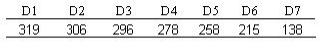Figure 8. Chart. Average deflections, FWD #131, August 12, 1998.

The normalized deflections are: 306/319 = .959, 296/319 = .928, etc. (Note that minor discrepancies may be due to rounding; the D1 deflection of 319 is really 319.220031738281, and all calculations used all available digits.)  These values are converted to absolute natural logarithms, and then raised separately to the powers 0.6 and 2.2. For example, for the third normalized deflection 0.9280, its natural logarithm is negative 0.0747, producing an absolute value of 0.0747, and the two powers of 0.6 and 2.2 of this number are 0.2109 and 0.0033. Then the first point, corresponding to the values for sensor 3 in the multiple linear regression, is determined according to the formula in figure 9.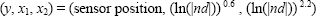Figure 9. Equation. y, x1, x2.

For the present example, the result is (12, 0.2109, 0.0033). Similar values are found for sensors 4, 5, and 6, and then a multiple linear regression is fit to find the values of a, b, and c to minimize the sums of squares, as shown in the equation given in figure 10.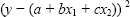Figure 10. Equation. a, b, c.

The spreadsheet calculations would then look like the data presented in table 9.

Table 9. Initial SLIC procedure calculations for sensor 7.
Sensor Mean Defl. Norm.Defl. |ln(nd)| |ln|(nd)^0.6 |ln|(nd)^2.2
1 319 1
2 306 0.959933 0.0409 0.1468854 0.0008822
3 296 0.927981 0.0747 0.2109341 0.0033256
4 278 0.871092 0.138 0.3047488 0.012817
5 258 0.808533 0.2125 0.3948721 0.0331391
6 215 0.673987 0.3945 0.5723452 0.1292447
7 138 0.432116 0.8391 0.9000702 0.679746

Recall that sensor 2 data weakens the accuracy of the prediction of sensor 7, on average, and that sensor 7 data would be left out of the model that predicts the position of sensor 7. So a multiple linear regression would be fit as shown in table 10:

Table 10. Final SLIC procedure calculations for sensor 7.
Sensor y x1 x2
3 12 0.2109341 0.0033256
4 18 0.3047488 0.012817
5 24 0.3948721 0.0331391
6 36 0.5723452 0.1292447

This was done, and the results are shown in table 11.

Regression Statistics Multiple R R Squared Adjusted R Squared 1 1 1 0.0662 4 ANOVA 2 314.99561 157.49781 #### 1 0.0043885 0.0043885 3 315 –1.549 0.2199251 –7.04254 0.09 64.021 0.8377741 76.418037 0.01 7.0493 2.233105 3.1567421 0.2

Then the values of a, b, and c can be (electronically) read from the output as –1.549, 64.021, and 7.0493, and the values of x1 and x2 for sensor 7 used to predict the position of sensor 7 as –1.549 + 64.021*.9000702 + 7.0493*0.679746. This value, 60.866, is the predicted value of the sensor 7 offset (in inches). This procedure was found to produce predictions that averaged close to 152.4 centimeters (cm) (60 inches) on datasets with no previously found error and with the least variation about this expected value. However, as can be seen in the graphs in appendices C through L, there is still some scatter, mostly due to the fact that an extrapolation somewhat far from the existing points is required. In the graphs that depict the time series of predictions of D7, found in appendices C through L, this prediction would correspond to exactly one point in graphs of D7 predictions for a particular FWD. The value of R2, in this case so close to 1 that it is rounded to 1, also would be graphed as one point in the appropriate graph of R2.

The entire procedure was repeated for sensor 2 predictions also, except that the model that best predicted 20.32 cm (8 inches) for sensor 2, was revised as shown in figure 11.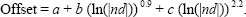Figure 11. Equation. Offset, sensor 2.

That is, only the value of d had to be changed, from 0.6 to 0.9, while the value of e remained at 2.2. As in the case of sensor 7, this work was based on a dataset believed to be error-free from previous work. The remainder of the procedure was identical, including the choice of an optimal set of data for predicting sensor 2, which also was the four closest sensors, sensors 3 through 6.